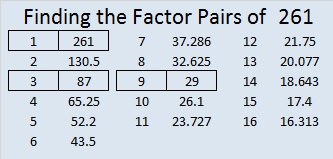# 261 and Level 2

• 261 is a composite number.
• Prime factorization: 261 = 3 x 3 x 29
• The exponents in the prime factorization are 2 and 1. Adding one to each and multiplying we get (2 + 1)(1 + 1) = 3 x 2 = 6. Therefore 261 has 6 factors.
• Factors of 261: 1, 3, 9, 29, 87, 261
• Factor pairs: 261 = 1 x 261, 3 x 87, 9 x 29
• Taking the factor pair with the largest square number factor, we get √261 = (√9)(√29) = 3√29 ≈ 16.155This puzzle will look like a multiplication table after you find the factors and then fill in the rest of the table.Print the puzzles or type the factors on this excel file: 10 Factors 2014-10-13This site uses Akismet to reduce spam. Learn how your comment data is processed.